# VLOOKUP Partial MatchArticle byJeevan A Y## What Is Partial Match With VLOOKUP?

VLOOKUP Partial Match is used to find information from a table using a partial match. This function is often used in the data analysis field. The VLOOKUP function retrieves values from another table based on the lookup value.

However, VLOOKUP requires the lookup value to be the same as in the lookup table; even a small space character on either table will result in a ‘not available’ error (#N/A).

To work efficiently with the VLOOKUP function, we should know that it can do the VLOOKUP partial match if the lookup value is combined with wildcard characters asterisk (*) in Excel. For instance, look at the following data.

In the main table A1:B7 (lookup table), we have a list of names and their brand value.

In cell C2, we need to fetch the brand value of the name ‘Sachin’ (lookup value). However, in the lookup table, we have the name ‘Sachin Tendulkar’ but the lookup value as ‘Sachin,’ i.e., only a partial name is available.

With the regular VLOOKUP function, we will get a #N/A error.

VLOOKUP looks for the exact match of the lookup value ‘Sachin’ but fails to find it in the main table range A1:B7. However, by combining the wildcard character asterisk, we can perform VLOOKUP partial match in the main table.

###### Key Takeaways
• VLOOKUP Partial Match returns the value for the first result in the table.
• Partial Match works only if the partial value is there in the lookup table.
• There are 3 types of wildcards in Excel, they are Asterisk (*), Question Mark (?), and Tilde (~).
• Asterisk (*) is used to match any number of characters in the word. For instance, “Wo*” will match the words Word, Work, Woke, Wonder, Wound, Woolen, etc…
• VLOOKUP fails to return if the partial value is there in the main table and the full value is there in the lookup value.

## How Does Partial Match In VOOKUP Work?

Before we understand how to perform VLOOKUP partial match, let us explain the wildcard character asterisk (*).

ASTERISK (*): This is the common and most used wildcard character in Excel. Asterisk (*) is used to match any number of characters in a word.

• Ex* = This can match the words that start with the characters “Ex” and ends with any number of characters i.e., Excellent, Excel, Experience, Extraordinary.
• *Ex = This can match the words that end with the characters “Ex” and starts with any number of characters i.e., Codex, FedEx, Cemex, Duplex, Vertex, Complex.
• *Ex* = This can match words that contain the character “Ex”. Starting and Ending of the words don’t matter here. i.e., Text, Lexes, Lexis, Dextrose.

Consider the following data in Excel to apply wildcard character with the Excel VLOOKUP function.

As we have discussed earlier, we have only the partial lookup value in cell D2. Let us use the asterisk (*) wildcard in Excel to perform a partial lookup.

1. As we have discussed earlier, we have only the partial lookup value in cell D2. Let us use the asterisk (*) wildcard to perform a partial lookup.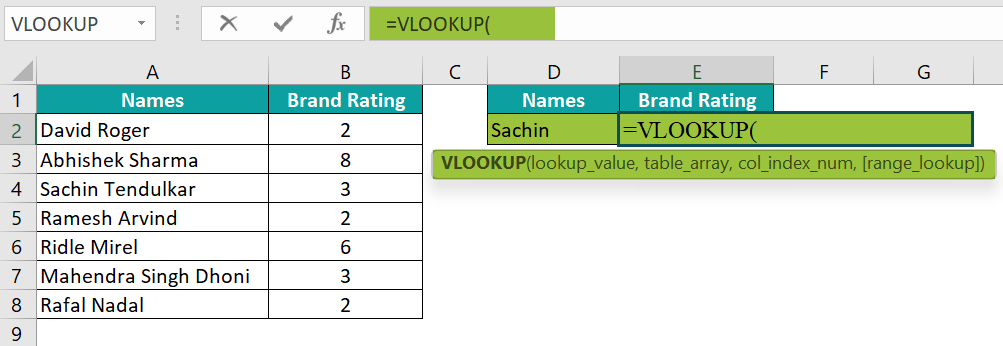2. Select the lookup value as cell D2.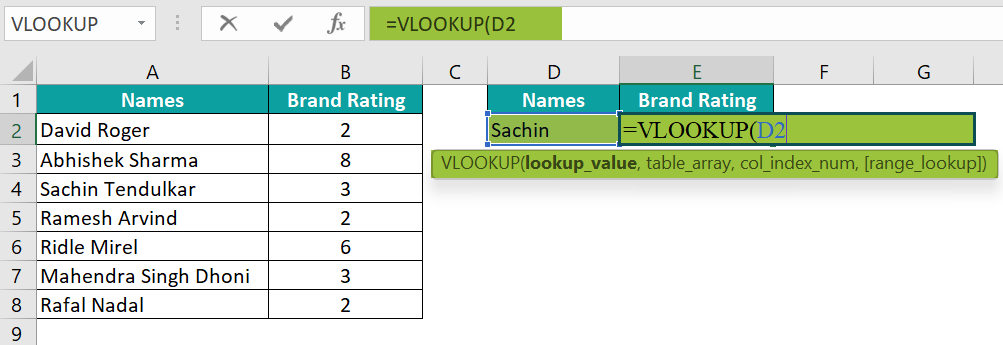3. As we know Sachin is the first name and we need to match the remaining characters after the first name. So, let us combine the wildcard character asterisk (*) by using the ampersand sign as shown in the following image.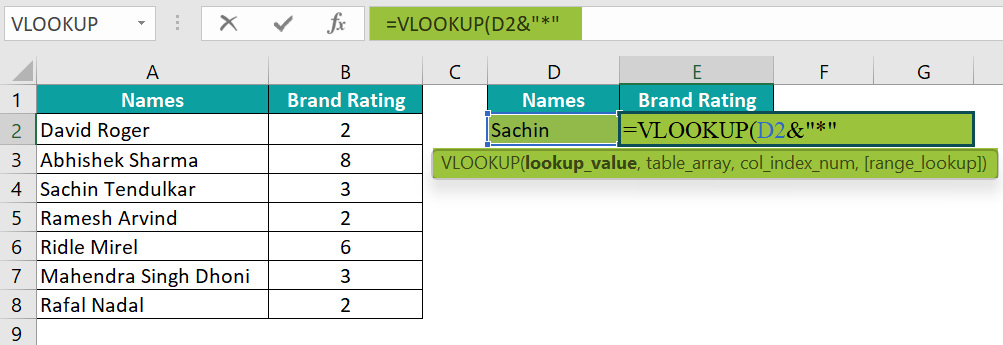4. Next choose the table array from A1:B8.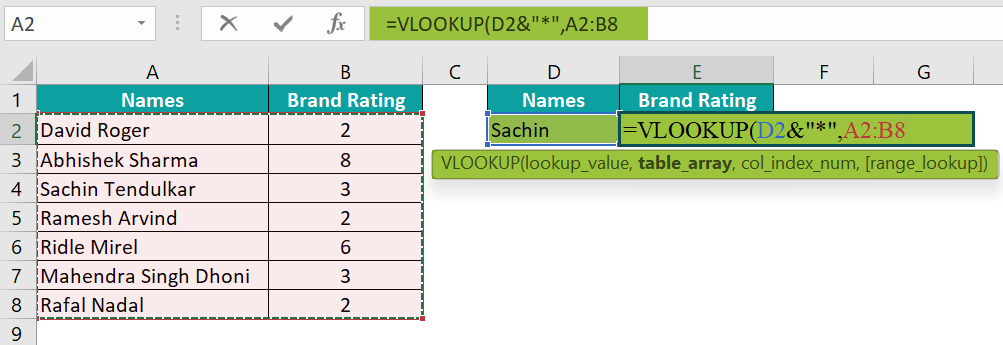5. Enter the column index number as 2 to fetch the brand rating column value.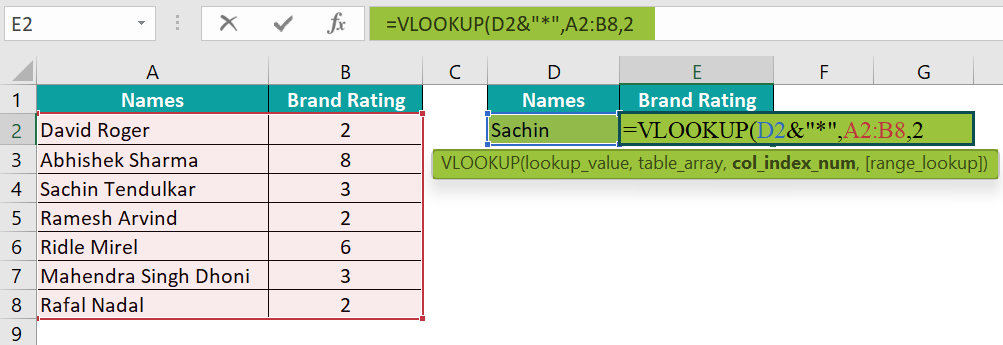6. Enter the match type as FALSE to get the exact match.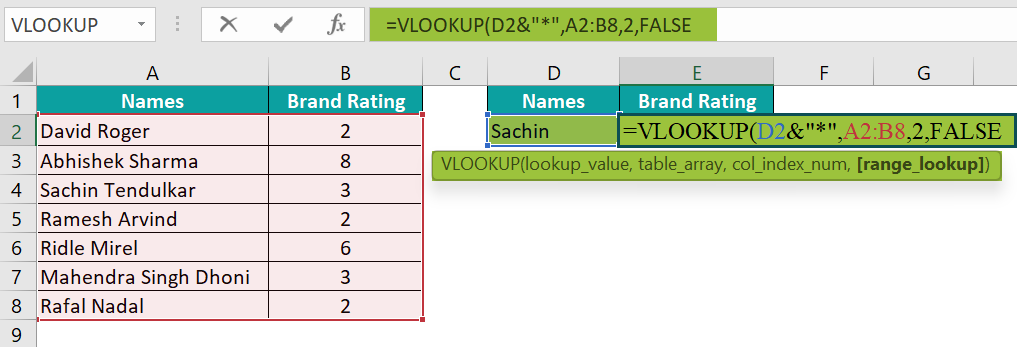7. Close the bracket and hit the Enter key to get the brand rating value for the name ‘Sachin.’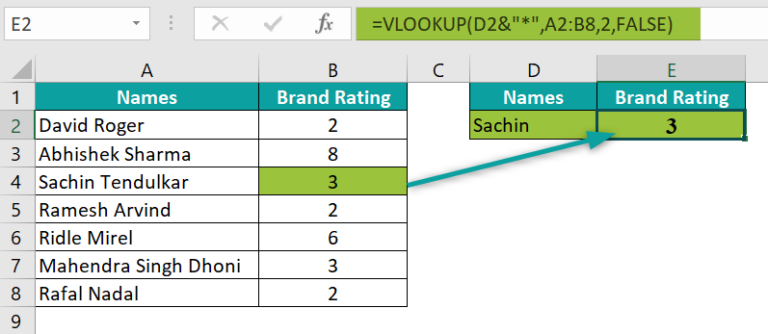There we go, even though we had a partial lookup value of ‘Sachin’ by combining the wildcard character asterisk (*) we are able to match the remaining character after the name ‘Sachin’ and retrieved the exact brand rating value from the main table.

### Examples

Let us understand the function with VLOOKUP Partial match example.

#### Example #1: Asterisk On Either Side Of Lookup Value

Let us continue with the same data from the above example.

This time we will use a different name ‘Singh’ as a VLOOKUP Partial match.

Let us apply the same logic as we have applied earlier in the above example.

Even though we have combined the wildcard character asterisk (*) after the lookup value, we ended up getting a #N/A error.

Let us closely examine what is causing this issue.

Sing&“*”: Here, the wildcard asterisk (*) is matching any number of characters after the word ‘Singh.’ But take look at the actual value in the main table.

The actual word is ‘Mahendra Singh Dhoni.’

Before the word ‘Singh’, there is another word, and this is not matched by the asterisk character. To match any number of characters on either side of the lookup value, we need to combine the asterisk (*) on either side of the lookup value ‘Singh.’

There we go, we have the result.

“*”&Singh&”*”: Here, the first asterisk character matches the word ‘Mahendra,’ and the second asterisk (*) character matches the word ‘Dhoni.’ Hence, we will end up getting the lookup value as ‘Mahendra Singh Dhoni’ which is exactly the same as in the main table.

#### Example #2: Possible Error With Partial Match With VLOOKUP

We need to know the possible errors and be cautious while applying the partial match up in VLOOKUP.

Let us learn the following data in Excel using VLOOKUP Partial match for this example.

We will try to find the invoice value for the company ‘Mojo’ in cell E2.

Let us apply the VLOOKUP function along with the wildcard character asterisk (*) to fetch the invoice value.

When the asterisk (*) is combined with the lookup value ‘Mojo’ it has found the partial match in the main table and matched the company name ‘Wallstreet Mojo Pvt Ltd’ and returned the invoice value as \$47,193.

However, when we closely look at the main table two companies have the word ‘Mojo’ in them.

• While dealing with these kinds of data we need to be absolutely sure of which company data we are trying to retrieve.
• In this example, the first matching value was ‘Wallstreet Mojo Pvt Ltd’ and VLOOLUP stopped the search right there. So returned the first matching value result as output.
• If we are really looking for the company ‘Xl Mojo Pvt Ltd’ invoice value this partial lookup value doesn’t work, and we will end up getting the wrong result.
• Now, let us look at another possible error. We will try to fetch the value for the company ‘Apple Inc.,’ using VLOOKUP Partial match.

The lookup value that we have used here is ‘Apple Inc.,’ along with the wildcard character. However, the result we get is an error.

The reason for this is in the main table company name is just ‘Apple’, but in the lookup value we have Apple Inc., So, partial lookup works only for the partial value of the lookup value not the partial value in the main table.

### Wildcard Character With LOOKUP

VLOOKUP function can be used with wildcard characters like an asterisk (*), question mark (*), and tilde (~).

#### Type #1: ASTERISK (*)

This is the common and most used wildcard in Excel. Asterisk (*) is used to match any number of characters in a word.

• Ex* = This can match the words that start with the characters “Ex” and ends with any number of characters i.e., Excellent, Excel, Experience, Extraordinary.
• *Ex = This can match the words that end with the characters “Ex” and starts with any number of characters i.e., Codex, FedEx, Cemex, Duplex, Vertex, Complex.
• *Ex* = This can match words that contain the character “Ex”. Starting and Ending of the words don’t matter here. i.e., Text, Lexes, Lexis, Dextrose.

#### Type #2: QUESTION MARK (?)

This wildcard represents any single character in the word.

• P?t = This can match the words that start with the word “P” and ends with the word “t” i.e., PAT, PUT, PET, POT.
• ??-?? = This will match the string of words i.e., ABC-CFF, DC-PC, A@#-S@#.

#### Type #3: TILDE (~)

This wildcard is used to find the wildcard characters in the word. Tilde (~) is used to cancel the wildcard presence.

• For instance, if we have the words Excellent, Excel, and if we need to find the exact word ‘Excel’ we generally put in the asterisk (*) wildcard character to find the partial match i.e., ‘Excel*’. However, this ends up searching both the words Excellent and Excel. To cancel the presence of the wildcard character asterisk (*) we can apply another wildcard character tilde (~) like the following one.

“Excel~*”

This will cancel the presence of the wildcard asterisk (*) and ends up searching only Excel in the list.

### Things To Remember

• VLOOKUP Partial Match may return wrong results in large datasets. Because if our lookup value is ‘Adam’ then it will treat ‘Adam Gilchrist’ and ‘Adam Smith’ as the same, so whichever comes first in the list will be treated as the matching result.
• Partial Lookup works if we have partial value only in the lookup value. If we have full value in the lookup cell and only partial value in the main table, the partial lookup will return a not available error (#N/A).
• Asterisk will match any number of characters before and after the lookup value if applied on either side of the lookup value.

1. Why is VLOOKUP partial match not working?

A VLOOKUP partial match will not work if the partial value is there in the lookup table and the full value is there in the lookup table.

2. How do I compare two Excel cells for VLOOKUP partial match?

If you are looking for VLOOKUP partial match based on two cells, then we need to combine both cells in the main table and then combine lookup value cells in the result cell.

3. How do VLOOKUP partial match multiple values?

For instance, let us understand the function with VLOOKUP Partial match example multiple values example.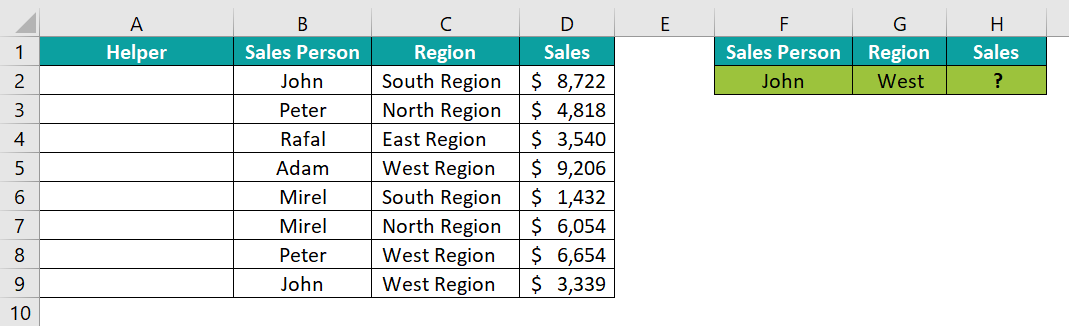We need to fetch the sales value based on the salesperson and region. However, when we look at the region we have only ‘West’ in the lookup value.
To perform these kinds of lookups first we need to combine both the columns in the main table as shown in the following image.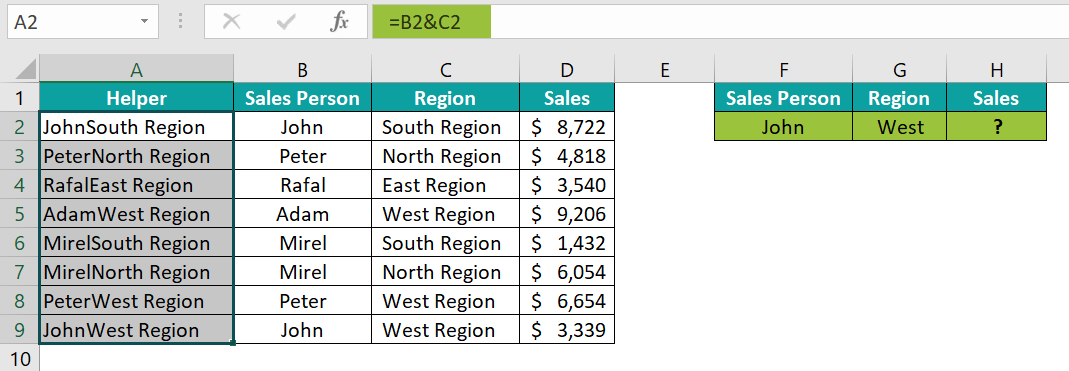Now apply VLOOKUP partial match as shown in the following image.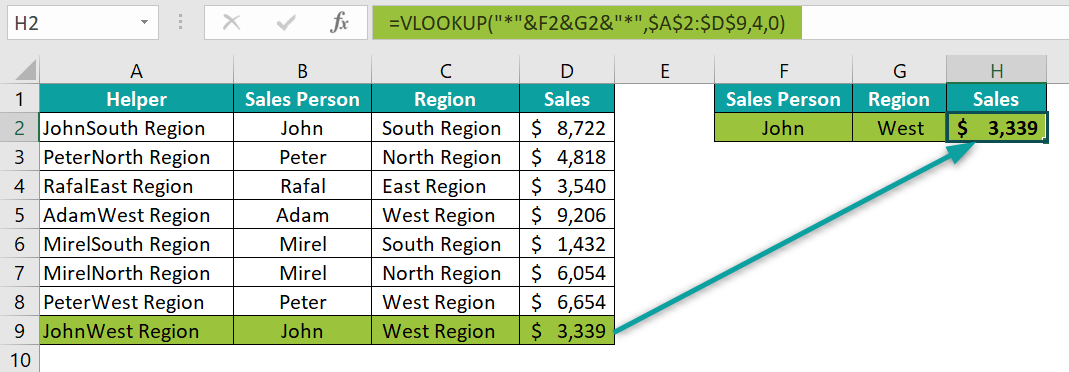It will match the lookup value partial for the sales person ‘John’ and for the region ‘West’ and retrieves the sales value as \$3,339.